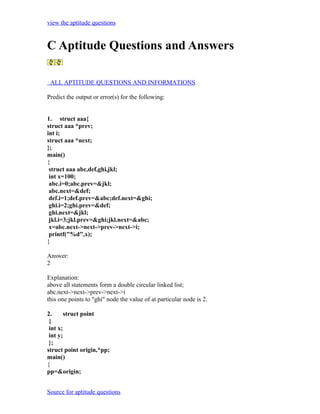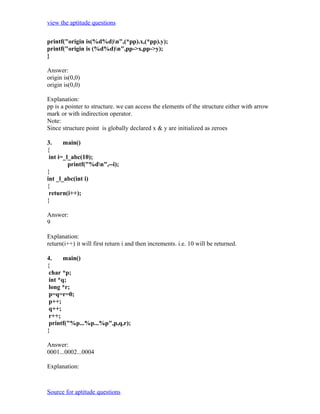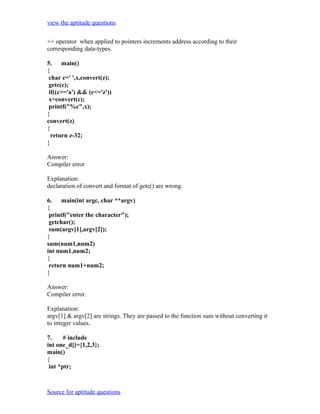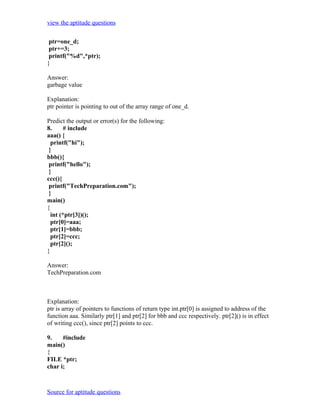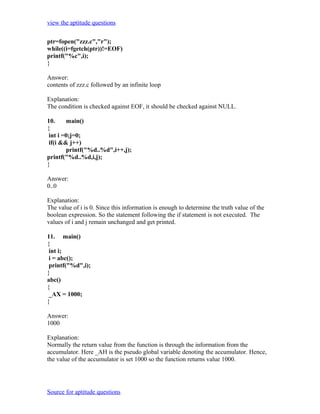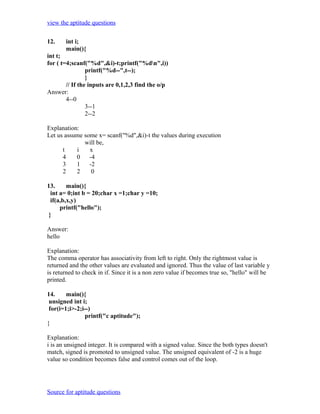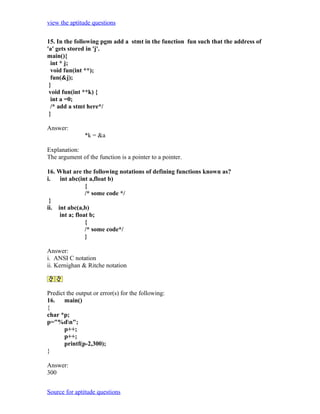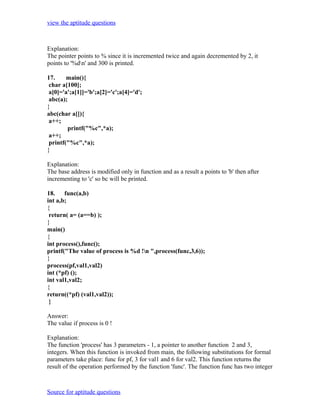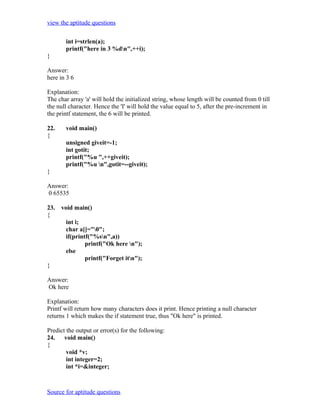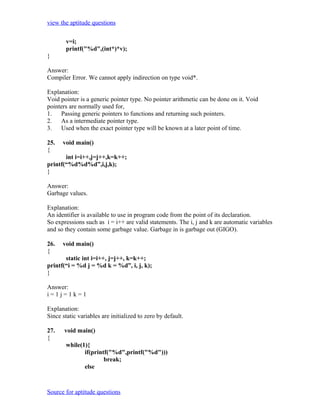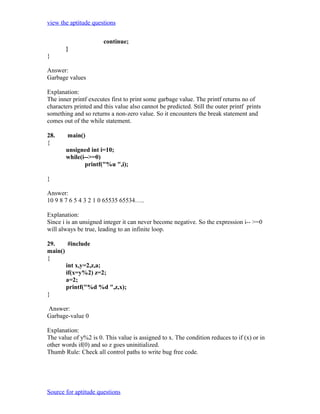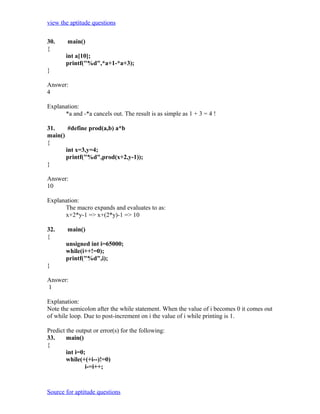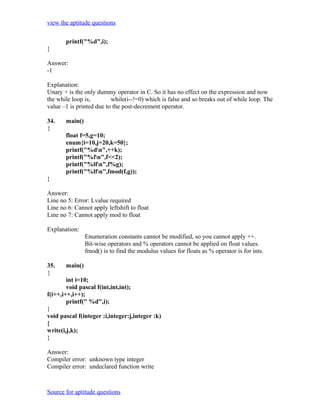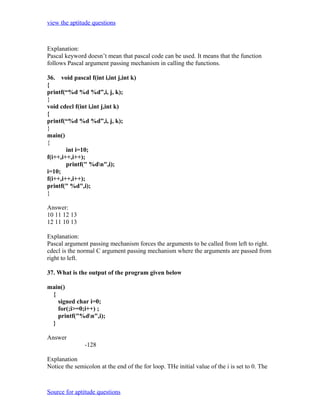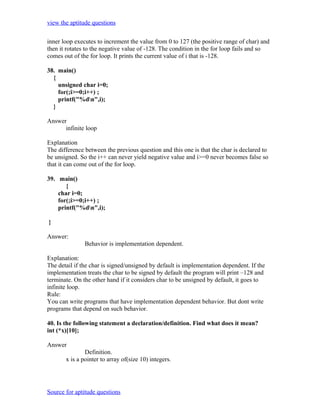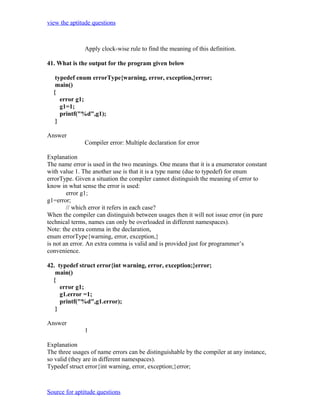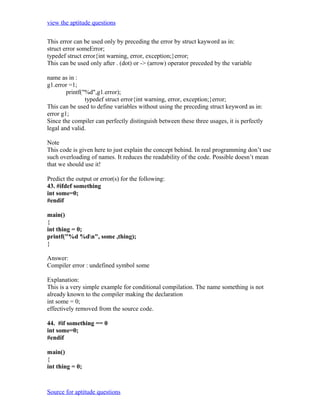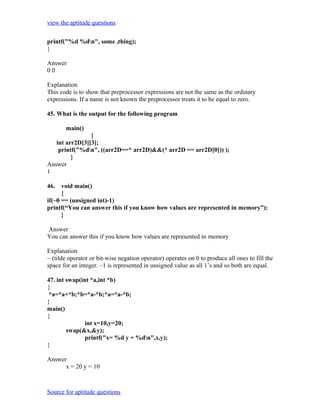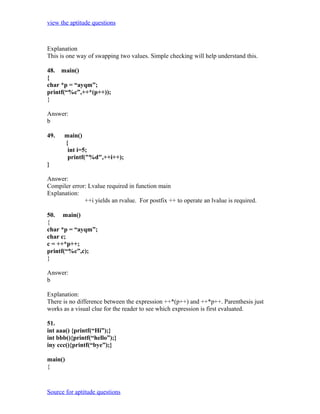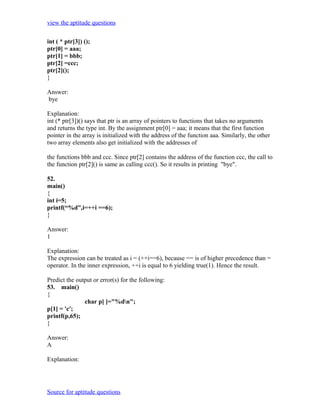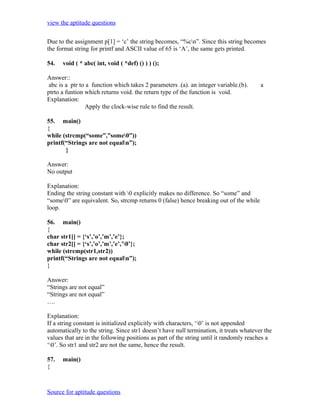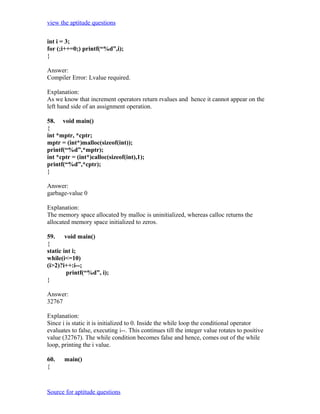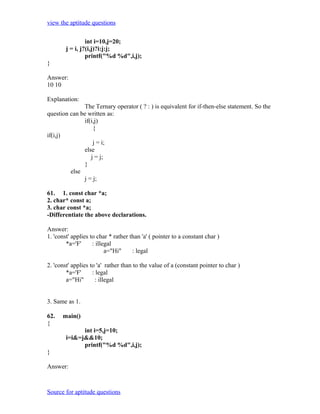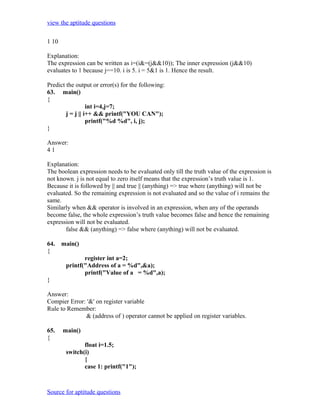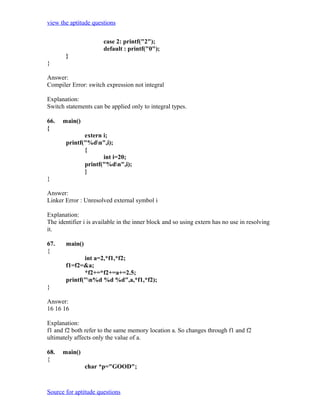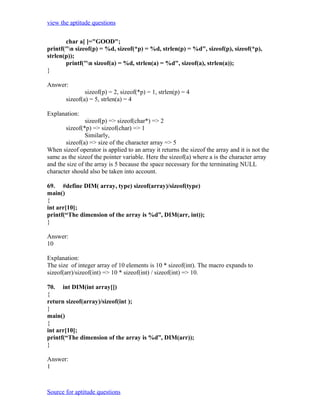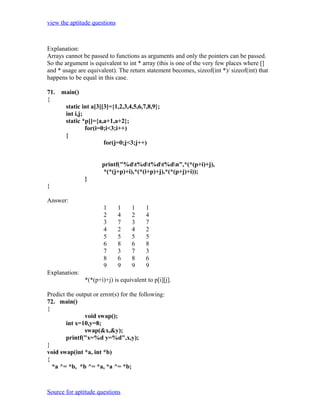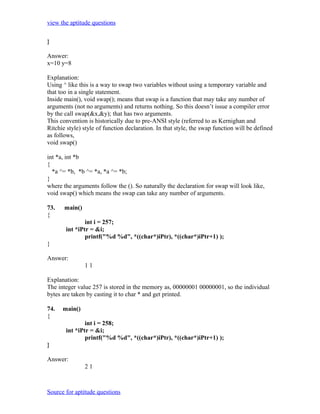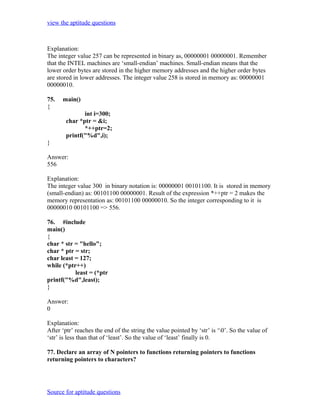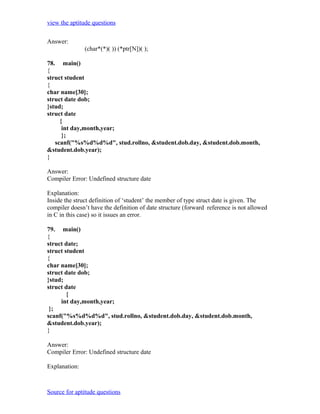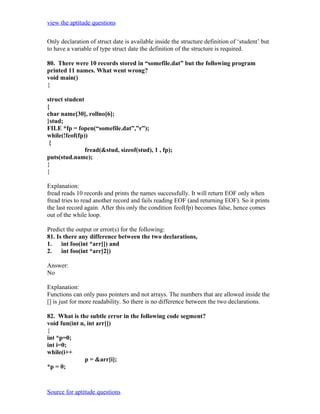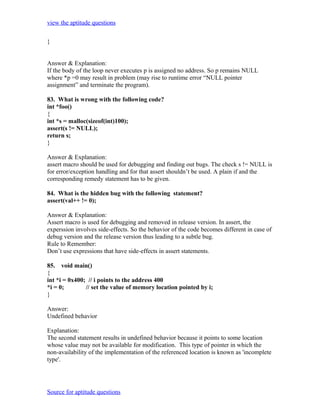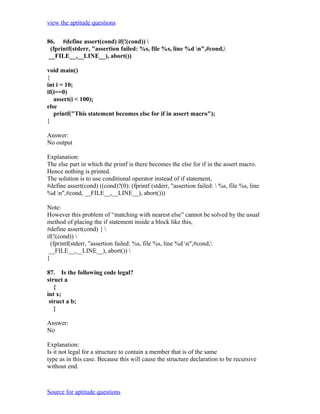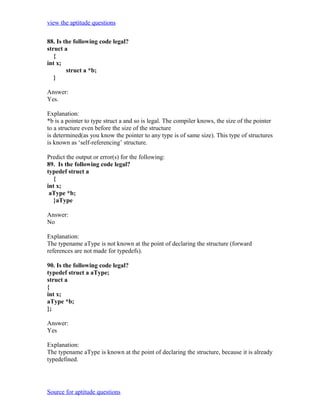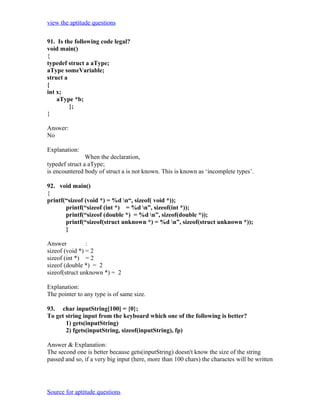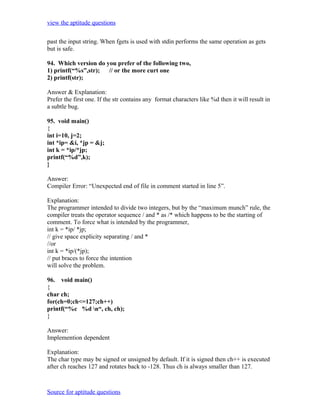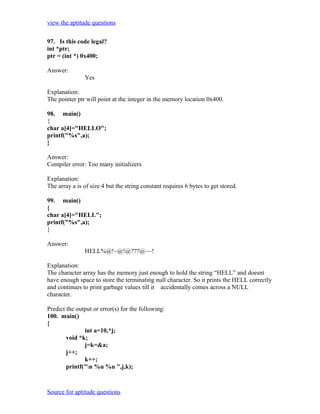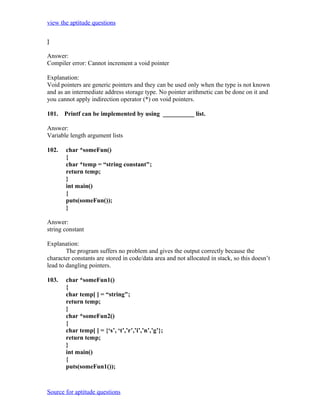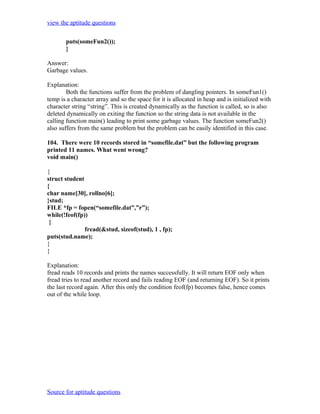Próximo SlideShare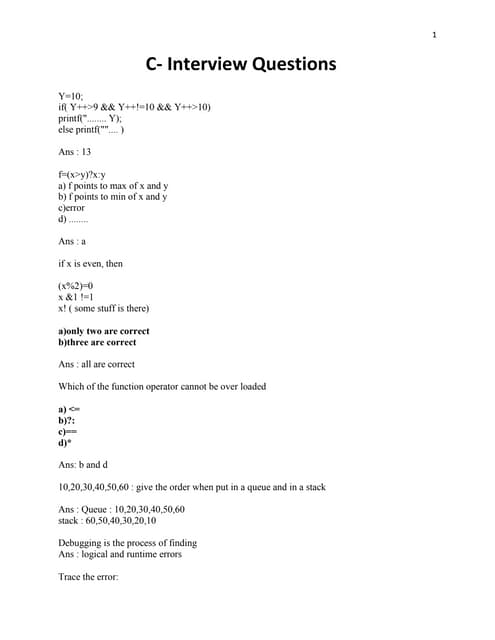C interview question answer 2
Cargando en ... 3
1 de 40

### C aptitude scribd

1. view the aptitude questions C Aptitude Questions and Answers ALL APTITUDE QUESTIONS AND INFORMATIONS Predict the output or error(s) for the following: 1. struct aaa{ struct aaa *prev; int i; struct aaa *next; }; main() { struct aaa abc,def,ghi,jkl; int x=100; abc.i=0;abc.prev=&jkl; abc.next=&def; def.i=1;def.prev=&abc;def.next=&ghi; ghi.i=2;ghi.prev=&def; ghi.next=&jkl; jkl.i=3;jkl.prev=&ghi;jkl.next=&abc; x=abc.next->next->prev->next->i; printf("%d",x); } Answer: 2 Explanation: above all statements form a double circular linked list; abc.next->next->prev->next->i this one points to "ghi" node the value of at particular node is 2. 2. struct point { int x; int y; }; struct point origin,*pp; main() { pp=&origin; Source for aptitude questions
2. view the aptitude questions printf("origin is(%d%d)n",(*pp).x,(*pp).y); printf("origin is (%d%d)n",pp->x,pp->y); } Answer: origin is(0,0) origin is(0,0) Explanation: pp is a pointer to structure. we can access the elements of the structure either with arrow mark or with indirection operator. Note: Since structure point is globally declared x & y are initialized as zeroes 3. main() { int i=_l_abc(10); printf("%dn",--i); } int _l_abc(int i) { return(i++); } Answer: 9 Explanation: return(i++) it will first return i and then increments. i.e. 10 will be returned. 4. main() { char *p; int *q; long *r; p=q=r=0; p++; q++; r++; printf("%p...%p...%p",p,q,r); } Answer: 0001...0002...0004 Explanation: Source for aptitude questions
3. view the aptitude questions ++ operator when applied to pointers increments address according to their corresponding data-types. 5. main() { char c=' ',x,convert(z); getc(c); if((c>='a') && (c<='z')) x=convert(c); printf("%c",x); } convert(z) { return z-32; } Answer: Compiler error Explanation: declaration of convert and format of getc() are wrong. 6. main(int argc, char **argv) { printf("enter the character"); getchar(); sum(argv,argv); } sum(num1,num2) int num1,num2; { return num1+num2; } Answer: Compiler error. Explanation: argv & argv are strings. They are passed to the function sum without converting it to integer values. 7. # include int one_d[]={1,2,3}; main() { int *ptr; Source for aptitude questions
4. view the aptitude questions ptr=one_d; ptr+=3; printf("%d",*ptr); } Answer: garbage value Explanation: ptr pointer is pointing to out of the array range of one_d. Predict the output or error(s) for the following: 8. # include aaa() { printf("hi"); } bbb(){ printf("hello"); } ccc(){ printf("TechPreparation.com"); } main() { int (*ptr)(); ptr=aaa; ptr=bbb; ptr=ccc; ptr(); } Answer: TechPreparation.com Explanation: ptr is array of pointers to functions of return type int.ptr is assigned to address of the function aaa. Similarly ptr and ptr for bbb and ccc respectively. ptr() is in effect of writing ccc(), since ptr points to ccc. 9. #include main() { FILE *ptr; char i; Source for aptitude questions
5. view the aptitude questions ptr=fopen("zzz.c","r"); while((i=fgetch(ptr))!=EOF) printf("%c",i); } Answer: contents of zzz.c followed by an infinite loop Explanation: The condition is checked against EOF, it should be checked against NULL. 10. main() { int i =0;j=0; if(i && j++) printf("%d..%d",i++,j); printf("%d..%d,i,j); } Answer: 0..0 Explanation: The value of i is 0. Since this information is enough to determine the truth value of the boolean expression. So the statement following the if statement is not executed. The values of i and j remain unchanged and get printed. 11. main() { int i; i = abc(); printf("%d",i); } abc() { _AX = 1000; } Answer: 1000 Explanation: Normally the return value from the function is through the information from the accumulator. Here _AH is the pseudo global variable denoting the accumulator. Hence, the value of the accumulator is set 1000 so the function returns value 1000. Source for aptitude questions
6. view the aptitude questions 12. int i; main(){ int t; for ( t=4;scanf("%d",&i)-t;printf("%dn",i)) printf("%d--",t--); } // If the inputs are 0,1,2,3 find the o/p Answer: 4--0 3--1 2--2 Explanation: Let us assume some x= scanf("%d",&i)-t the values during execution will be, t i x 4 0 -4 3 1 -2 2 2 0 13. main(){ int a= 0;int b = 20;char x =1;char y =10; if(a,b,x,y) printf("hello"); } Answer: hello Explanation: The comma operator has associativity from left to right. Only the rightmost value is returned and the other values are evaluated and ignored. Thus the value of last variable y is returned to check in if. Since it is a non zero value if becomes true so, "hello" will be printed. 14. main(){ unsigned int i; for(i=1;i>-2;i--) printf("c aptitude"); } Explanation: i is an unsigned integer. It is compared with a signed value. Since the both types doesn't match, signed is promoted to unsigned value. The unsigned equivalent of -2 is a huge value so condition becomes false and control comes out of the loop. Source for aptitude questions
7. view the aptitude questions 15. In the following pgm add a stmt in the function fun such that the address of 'a' gets stored in 'j'. main(){ int * j; void fun(int **); fun(&j); } void fun(int **k) { int a =0; /* add a stmt here*/ } Answer: *k = &a Explanation: The argument of the function is a pointer to a pointer. 16. What are the following notations of defining functions known as? i. int abc(int a,float b) { /* some code */ } ii. int abc(a,b) int a; float b; { /* some code*/ } Answer: i. ANSI C notation ii. Kernighan & Ritche notation Predict the output or error(s) for the following: 16. main() { char *p; p="%dn"; p++; p++; printf(p-2,300); } Answer: 300 Source for aptitude questions
8. view the aptitude questions Explanation: The pointer points to % since it is incremented twice and again decremented by 2, it points to '%dn' and 300 is printed. 17. main(){ char a; a='a';a]='b';a='c';a='d'; abc(a); } abc(char a[]){ a++; printf("%c",*a); a++; printf("%c",*a); } Explanation: The base address is modified only in function and as a result a points to 'b' then after incrementing to 'c' so bc will be printed. 18. func(a,b) int a,b; { return( a= (a==b) ); } main() { int process(),func(); printf("The value of process is %d !n ",process(func,3,6)); } process(pf,val1,val2) int (*pf) (); int val1,val2; { return((*pf) (val1,val2)); } Answer: The value if process is 0 ! Explanation: The function 'process' has 3 parameters - 1, a pointer to another function 2 and 3, integers. When this function is invoked from main, the following substitutions for formal parameters take place: func for pf, 3 for val1 and 6 for val2. This function returns the result of the operation performed by the function 'func'. The function func has two integer Source for aptitude questions
9. view the aptitude questions parameters. The formal parameters are substituted as 3 for a and 6 for b. since 3 is not equal to 6, a==b returns 0. therefore the function returns 0 which in turn is returned by the function 'process'. 19. void main() { static int i=5; if(--i){ main(); printf("%d ",i); } } Answer: 0000 Explanation: The variable "I" is declared as static, hence memory for I will be allocated for only once, as it encounters the statement. The function main() will be called recursively unless I becomes equal to 0, and since main() is recursively called, so the value of static I ie., 0 will be printed every time the control is returned. 20. void main() { int k=ret(sizeof(float)); printf("n here value is %d",++k); } int ret(int ret) { ret += 2.5; return(ret); } Answer: Here value is 7 Explanation: The int ret(int ret), ie., the function name and the argument name can be the same. Firstly, the function ret() is called in which the sizeof(float) ie., 4 is passed, after the first expression the value in ret will be 6, as ret is integer hence the value stored in ret will have implicit type conversion from float to int. The ret is returned in main() it is printed after and preincrement. 21. void main() { char a[]="123450"; Source for aptitude questions
10. view the aptitude questions int i=strlen(a); printf("here in 3 %dn",++i); } Answer: here in 3 6 Explanation: The char array 'a' will hold the initialized string, whose length will be counted from 0 till the null character. Hence the 'I' will hold the value equal to 5, after the pre-increment in the printf statement, the 6 will be printed. 22. void main() { unsigned giveit=-1; int gotit; printf("%u ",++giveit); printf("%u n",gotit=--giveit); } Answer: 0 65535 23. void main() { int i; char a[]="0"; if(printf("%sn",a)) printf("Ok here n"); else printf("Forget itn"); } Answer: Ok here Explanation: Printf will return how many characters does it print. Hence printing a null character returns 1 which makes the if statement true, thus "Ok here" is printed. Predict the output or error(s) for the following: 24. void main() { void *v; int integer=2; int *i=&integer; Source for aptitude questions
11. view the aptitude questions v=i; printf("%d",(int*)*v); } Answer: Compiler Error. We cannot apply indirection on type void*. Explanation: Void pointer is a generic pointer type. No pointer arithmetic can be done on it. Void pointers are normally used for, 1. Passing generic pointers to functions and returning such pointers. 2. As a intermediate pointer type. 3. Used when the exact pointer type will be known at a later point of time. 25. void main() { int i=i++,j=j++,k=k++; printf(“%d%d%d”,i,j,k); } Answer: Garbage values. Explanation: An identifier is available to use in program code from the point of its declaration. So expressions such as i = i++ are valid statements. The i, j and k are automatic variables and so they contain some garbage value. Garbage in is garbage out (GIGO). 26. void main() { static int i=i++, j=j++, k=k++; printf(“i = %d j = %d k = %d”, i, j, k); } Answer: i=1j=1k=1 Explanation: Since static variables are initialized to zero by default. 27. void main() { while(1){ if(printf("%d",printf("%d"))) break; else Source for aptitude questions
12. view the aptitude questions continue; } } Answer: Garbage values Explanation: The inner printf executes first to print some garbage value. The printf returns no of characters printed and this value also cannot be predicted. Still the outer printf prints something and so returns a non-zero value. So it encounters the break statement and comes out of the while statement. 28. main() { unsigned int i=10; while(i-->=0) printf("%u ",i); } Answer: 10 9 8 7 6 5 4 3 2 1 0 65535 65534….. Explanation: Since i is an unsigned integer it can never become negative. So the expression i-- >=0 will always be true, leading to an infinite loop. 29. #include main() { int x,y=2,z,a; if(x=y%2) z=2; a=2; printf("%d %d ",z,x); } Answer: Garbage-value 0 Explanation: The value of y%2 is 0. This value is assigned to x. The condition reduces to if (x) or in other words if(0) and so z goes uninitialized. Thumb Rule: Check all control paths to write bug free code. Source for aptitude questions
13. view the aptitude questions 30. main() { int a; printf("%d",*a+1-*a+3); } Answer: 4 Explanation: *a and -*a cancels out. The result is as simple as 1 + 3 = 4 ! 31. #define prod(a,b) a*b main() { int x=3,y=4; printf("%d",prod(x+2,y-1)); } Answer: 10 Explanation: The macro expands and evaluates to as: x+2*y-1 => x+(2*y)-1 => 10 32. main() { unsigned int i=65000; while(i++!=0); printf("%d",i); } Answer: 1 Explanation: Note the semicolon after the while statement. When the value of i becomes 0 it comes out of while loop. Due to post-increment on i the value of i while printing is 1. Predict the output or error(s) for the following: 33. main() { int i=0; while(+(+i--)!=0) i-=i++; Source for aptitude questions
14. view the aptitude questions printf("%d",i); } Answer: -1 Explanation: Unary + is the only dummy operator in C. So it has no effect on the expression and now the while loop is, while(i--!=0) which is false and so breaks out of while loop. The value –1 is printed due to the post-decrement operator. 34. main() { float f=5,g=10; enum{i=10,j=20,k=50}; printf("%dn",++k); printf("%fn",f<<2); printf("%lfn",f%g); printf("%lfn",fmod(f,g)); } Answer: Line no 5: Error: Lvalue required Line no 6: Cannot apply leftshift to float Line no 7: Cannot apply mod to float Explanation: Enumeration constants cannot be modified, so you cannot apply ++. Bit-wise operators and % operators cannot be applied on float values. fmod() is to find the modulus values for floats as % operator is for ints. 35. main() { int i=10; void pascal f(int,int,int); f(i++,i++,i++); printf(" %d",i); } void pascal f(integer :i,integer:j,integer :k) { write(i,j,k); } Answer: Compiler error: unknown type integer Compiler error: undeclared function write Source for aptitude questions
15. view the aptitude questions Explanation: Pascal keyword doesn’t mean that pascal code can be used. It means that the function follows Pascal argument passing mechanism in calling the functions. 36. void pascal f(int i,int j,int k) { printf(“%d %d %d”,i, j, k); } void cdecl f(int i,int j,int k) { printf(“%d %d %d”,i, j, k); } main() { int i=10; f(i++,i++,i++); printf(" %dn",i); i=10; f(i++,i++,i++); printf(" %d",i); } Answer: 10 11 12 13 12 11 10 13 Explanation: Pascal argument passing mechanism forces the arguments to be called from left to right. cdecl is the normal C argument passing mechanism where the arguments are passed from right to left. 37. What is the output of the program given below main() { signed char i=0; for(;i>=0;i++) ; printf("%dn",i); } Answer -128 Explanation Notice the semicolon at the end of the for loop. THe initial value of the i is set to 0. The Source for aptitude questions
16. view the aptitude questions inner loop executes to increment the value from 0 to 127 (the positive range of char) and then it rotates to the negative value of -128. The condition in the for loop fails and so comes out of the for loop. It prints the current value of i that is -128. 38. main() { unsigned char i=0; for(;i>=0;i++) ; printf("%dn",i); } Answer infinite loop Explanation The difference between the previous question and this one is that the char is declared to be unsigned. So the i++ can never yield negative value and i>=0 never becomes false so that it can come out of the for loop. 39. main() { char i=0; for(;i>=0;i++) ; printf("%dn",i); } Answer: Behavior is implementation dependent. Explanation: The detail if the char is signed/unsigned by default is implementation dependent. If the implementation treats the char to be signed by default the program will print –128 and terminate. On the other hand if it considers char to be unsigned by default, it goes to infinite loop. Rule: You can write programs that have implementation dependent behavior. But dont write programs that depend on such behavior. 40. Is the following statement a declaration/definition. Find what does it mean? int (*x); Answer Definition. x is a pointer to array of(size 10) integers. Source for aptitude questions
17. view the aptitude questions Apply clock-wise rule to find the meaning of this definition. 41. What is the output for the program given below typedef enum errorType{warning, error, exception,}error; main() { error g1; g1=1; printf("%d",g1); } Answer Compiler error: Multiple declaration for error Explanation The name error is used in the two meanings. One means that it is a enumerator constant with value 1. The another use is that it is a type name (due to typedef) for enum errorType. Given a situation the compiler cannot distinguish the meaning of error to know in what sense the error is used: error g1; g1=error; // which error it refers in each case? When the compiler can distinguish between usages then it will not issue error (in pure technical terms, names can only be overloaded in different namespaces). Note: the extra comma in the declaration, enum errorType{warning, error, exception,} is not an error. An extra comma is valid and is provided just for programmer’s convenience. 42. typedef struct error{int warning, error, exception;}error; main() { error g1; g1.error =1; printf("%d",g1.error); } Answer 1 Explanation The three usages of name errors can be distinguishable by the compiler at any instance, so valid (they are in different namespaces). Typedef struct error{int warning, error, exception;}error; Source for aptitude questions
18. view the aptitude questions This error can be used only by preceding the error by struct kayword as in: struct error someError; typedef struct error{int warning, error, exception;}error; This can be used only after . (dot) or -> (arrow) operator preceded by the variable name as in : g1.error =1; printf("%d",g1.error); typedef struct error{int warning, error, exception;}error; This can be used to define variables without using the preceding struct keyword as in: error g1; Since the compiler can perfectly distinguish between these three usages, it is perfectly legal and valid. Note This code is given here to just explain the concept behind. In real programming don’t use such overloading of names. It reduces the readability of the code. Possible doesn’t mean that we should use it! Predict the output or error(s) for the following: 43. #ifdef something int some=0; #endif main() { int thing = 0; printf("%d %dn", some ,thing); } Answer: Compiler error : undefined symbol some Explanation: This is a very simple example for conditional compilation. The name something is not already known to the compiler making the declaration int some = 0; effectively removed from the source code. 44. #if something == 0 int some=0; #endif main() { int thing = 0; Source for aptitude questions
19. view the aptitude questions printf("%d %dn", some ,thing); } Answer 00 Explanation This code is to show that preprocessor expressions are not the same as the ordinary expressions. If a name is not known the preprocessor treats it to be equal to zero. 45. What is the output for the following program main() { int arr2D; printf("%dn", ((arr2D==* arr2D)&&(* arr2D == arr2D)) ); } Answer 1 46. void main() { if(~0 == (unsigned int)-1) printf(“You can answer this if you know how values are represented in memory”); } Answer You can answer this if you know how values are represented in memory Explanation ~ (tilde operator or bit-wise negation operator) operates on 0 to produce all ones to fill the space for an integer. –1 is represented in unsigned value as all 1’s and so both are equal. 47. int swap(int *a,int *b) { *a=*a+*b;*b=*a-*b;*a=*a-*b; } main() { int x=10,y=20; swap(&x,&y); printf("x= %d y = %dn",x,y); } Answer x = 20 y = 10 Source for aptitude questions
20. view the aptitude questions Explanation This is one way of swapping two values. Simple checking will help understand this. 48. main() { char *p = “ayqm”; printf(“%c”,++*(p++)); } Answer: b 49. main() { int i=5; printf("%d",++i++); } Answer: Compiler error: Lvalue required in function main Explanation: ++i yields an rvalue. For postfix ++ to operate an lvalue is required. 50. main() { char *p = “ayqm”; char c; c = ++*p++; printf(“%c”,c); } Answer: b Explanation: There is no difference between the expression ++*(p++) and ++*p++. Parenthesis just works as a visual clue for the reader to see which expression is first evaluated. 51. int aaa() {printf(“Hi”);} int bbb(){printf(“hello”);} iny ccc(){printf(“bye”);} main() { Source for aptitude questions
21. view the aptitude questions int ( * ptr) (); ptr = aaa; ptr = bbb; ptr =ccc; ptr(); } Answer: bye Explanation: int (* ptr)() says that ptr is an array of pointers to functions that takes no arguments and returns the type int. By the assignment ptr = aaa; it means that the first function pointer in the array is initialized with the address of the function aaa. Similarly, the other two array elements also get initialized with the addresses of the functions bbb and ccc. Since ptr contains the address of the function ccc, the call to the function ptr() is same as calling ccc(). So it results in printing "bye". 52. main() { int i=5; printf(“%d”,i=++i ==6); } Answer: 1 Explanation: The expression can be treated as i = (++i==6), because == is of higher precedence than = operator. In the inner expression, ++i is equal to 6 yielding true(1). Hence the result. Predict the output or error(s) for the following: 53. main() { char p[ ]="%dn"; p = 'c'; printf(p,65); } Answer: A Explanation: Source for aptitude questions
22. view the aptitude questions Due to the assignment p = ‘c’ the string becomes, “%cn”. Since this string becomes the format string for printf and ASCII value of 65 is ‘A’, the same gets printed. 54. void ( * abc( int, void ( *def) () ) ) (); Answer:: abc is a ptr to a function which takes 2 parameters .(a). an integer variable.(b). a ptrto a funtion which returns void. the return type of the function is void. Explanation: Apply the clock-wise rule to find the result. 55. main() { while (strcmp(“some”,”some0”)) printf(“Strings are not equaln”); } Answer: No output Explanation: Ending the string constant with 0 explicitly makes no difference. So “some” and “some0” are equivalent. So, strcmp returns 0 (false) hence breaking out of the while loop. 56. main() { char str1[] = {‘s’,’o’,’m’,’e’}; char str2[] = {‘s’,’o’,’m’,’e’,’0’}; while (strcmp(str1,str2)) printf(“Strings are not equaln”); } Answer: “Strings are not equal” “Strings are not equal” …. Explanation: If a string constant is initialized explicitly with characters, ‘0’ is not appended automatically to the string. Since str1 doesn’t have null termination, it treats whatever the values that are in the following positions as part of the string until it randomly reaches a ‘0’. So str1 and str2 are not the same, hence the result. 57. main() { Source for aptitude questions
23. view the aptitude questions int i = 3; for (;i++=0;) printf(“%d”,i); } Answer: Compiler Error: Lvalue required. Explanation: As we know that increment operators return rvalues and hence it cannot appear on the left hand side of an assignment operation. 58. void main() { int *mptr, *cptr; mptr = (int*)malloc(sizeof(int)); printf(“%d”,*mptr); int *cptr = (int*)calloc(sizeof(int),1); printf(“%d”,*cptr); } Answer: garbage-value 0 Explanation: The memory space allocated by malloc is uninitialized, whereas calloc returns the allocated memory space initialized to zeros. 59. void main() { static int i; while(i<=10) (i>2)?i++:i--; printf(“%d”, i); } Answer: 32767 Explanation: Since i is static it is initialized to 0. Inside the while loop the conditional operator evaluates to false, executing i--. This continues till the integer value rotates to positive value (32767). The while condition becomes false and hence, comes out of the while loop, printing the i value. 60. main() { Source for aptitude questions
24. view the aptitude questions int i=10,j=20; j = i, j?(i,j)?i:j:j; printf("%d %d",i,j); } Answer: 10 10 Explanation: The Ternary operator ( ? : ) is equivalent for if-then-else statement. So the question can be written as: if(i,j) { if(i,j) j = i; else j = j; } else j = j; 61. 1. const char *a; 2. char* const a; 3. char const *a; -Differentiate the above declarations. Answer: 1. 'const' applies to char * rather than 'a' ( pointer to a constant char ) *a='F' : illegal a="Hi" : legal 2. 'const' applies to 'a' rather than to the value of a (constant pointer to char ) *a='F' : legal a="Hi" : illegal 3. Same as 1. 62. main() { int i=5,j=10; i=i&=j&&10; printf("%d %d",i,j); } Answer: Source for aptitude questions
25. view the aptitude questions 1 10 Explanation: The expression can be written as i=(i&=(j&&10)); The inner expression (j&&10) evaluates to 1 because j==10. i is 5. i = 5&1 is 1. Hence the result. Predict the output or error(s) for the following: 63. main() { int i=4,j=7; j = j || i++ && printf("YOU CAN"); printf("%d %d", i, j); } Answer: 41 Explanation: The boolean expression needs to be evaluated only till the truth value of the expression is not known. j is not equal to zero itself means that the expression’s truth value is 1. Because it is followed by || and true || (anything) => true where (anything) will not be evaluated. So the remaining expression is not evaluated and so the value of i remains the same. Similarly when && operator is involved in an expression, when any of the operands become false, the whole expression’s truth value becomes false and hence the remaining expression will not be evaluated. false && (anything) => false where (anything) will not be evaluated. 64. main() { register int a=2; printf("Address of a = %d",&a); printf("Value of a = %d",a); } Answer: Compier Error: '&' on register variable Rule to Remember: & (address of ) operator cannot be applied on register variables. 65. main() { float i=1.5; switch(i) { case 1: printf("1"); Source for aptitude questions
26. view the aptitude questions case 2: printf("2"); default : printf("0"); } } Answer: Compiler Error: switch expression not integral Explanation: Switch statements can be applied only to integral types. 66. main() { extern i; printf("%dn",i); { int i=20; printf("%dn",i); } } Answer: Linker Error : Unresolved external symbol i Explanation: The identifier i is available in the inner block and so using extern has no use in resolving it. 67. main() { int a=2,*f1,*f2; f1=f2=&a; *f2+=*f2+=a+=2.5; printf("n%d %d %d",a,*f1,*f2); } Answer: 16 16 16 Explanation: f1 and f2 both refer to the same memory location a. So changes through f1 and f2 ultimately affects only the value of a. 68. main() { char *p="GOOD"; Source for aptitude questions
27. view the aptitude questions char a[ ]="GOOD"; printf("n sizeof(p) = %d, sizeof(*p) = %d, strlen(p) = %d", sizeof(p), sizeof(*p), strlen(p)); printf("n sizeof(a) = %d, strlen(a) = %d", sizeof(a), strlen(a)); } Answer: sizeof(p) = 2, sizeof(*p) = 1, strlen(p) = 4 sizeof(a) = 5, strlen(a) = 4 Explanation: sizeof(p) => sizeof(char*) => 2 sizeof(*p) => sizeof(char) => 1 Similarly, sizeof(a) => size of the character array => 5 When sizeof operator is applied to an array it returns the sizeof the array and it is not the same as the sizeof the pointer variable. Here the sizeof(a) where a is the character array and the size of the array is 5 because the space necessary for the terminating NULL character should also be taken into account. 69. #define DIM( array, type) sizeof(array)/sizeof(type) main() { int arr; printf(“The dimension of the array is %d”, DIM(arr, int)); } Answer: 10 Explanation: The size of integer array of 10 elements is 10 * sizeof(int). The macro expands to sizeof(arr)/sizeof(int) => 10 * sizeof(int) / sizeof(int) => 10. 70. int DIM(int array[]) { return sizeof(array)/sizeof(int ); } main() { int arr; printf(“The dimension of the array is %d”, DIM(arr)); } Answer: 1 Source for aptitude questions
28. view the aptitude questions Explanation: Arrays cannot be passed to functions as arguments and only the pointers can be passed. So the argument is equivalent to int * array (this is one of the very few places where [] and * usage are equivalent). The return statement becomes, sizeof(int *)/ sizeof(int) that happens to be equal in this case. 71. main() { static int a={1,2,3,4,5,6,7,8,9}; int i,j; static *p[]={a,a+1,a+2}; for(i=0;i<3;i++) { for(j=0;j<3;j++) printf("%dt%dt%dt%dn",*(*(p+i)+j), *(*(j+p)+i),*(*(i+p)+j),*(*(p+j)+i)); } } Answer: 1 1 1 1 2 4 2 4 3 7 3 7 4 2 4 2 5 5 5 5 6 8 6 8 7 3 7 3 8 6 8 6 9 9 9 9 Explanation: *(*(p+i)+j) is equivalent to p[i][j]. Predict the output or error(s) for the following: 72. main() { void swap(); int x=10,y=8; swap(&x,&y); printf("x=%d y=%d",x,y); } void swap(int *a, int *b) { *a ^= *b, *b ^= *a, *a ^= *b; Source for aptitude questions
29. view the aptitude questions } Answer: x=10 y=8 Explanation: Using ^ like this is a way to swap two variables without using a temporary variable and that too in a single statement. Inside main(), void swap(); means that swap is a function that may take any number of arguments (not no arguments) and returns nothing. So this doesn’t issue a compiler error by the call swap(&x,&y); that has two arguments. This convention is historically due to pre-ANSI style (referred to as Kernighan and Ritchie style) style of function declaration. In that style, the swap function will be defined as follows, void swap() int *a, int *b { *a ^= *b, *b ^= *a, *a ^= *b; } where the arguments follow the (). So naturally the declaration for swap will look like, void swap() which means the swap can take any number of arguments. 73. main() { int i = 257; int *iPtr = &i; printf("%d %d", *((char*)iPtr), *((char*)iPtr+1) ); } Answer: 11 Explanation: The integer value 257 is stored in the memory as, 00000001 00000001, so the individual bytes are taken by casting it to char * and get printed. 74. main() { int i = 258; int *iPtr = &i; printf("%d %d", *((char*)iPtr), *((char*)iPtr+1) ); } Answer: 21 Source for aptitude questions
30. view the aptitude questions Explanation: The integer value 257 can be represented in binary as, 00000001 00000001. Remember that the INTEL machines are ‘small-endian’ machines. Small-endian means that the lower order bytes are stored in the higher memory addresses and the higher order bytes are stored in lower addresses. The integer value 258 is stored in memory as: 00000001 00000010. 75. main() { int i=300; char *ptr = &i; *++ptr=2; printf("%d",i); } Answer: 556 Explanation: The integer value 300 in binary notation is: 00000001 00101100. It is stored in memory (small-endian) as: 00101100 00000001. Result of the expression *++ptr = 2 makes the memory representation as: 00101100 00000010. So the integer corresponding to it is 00000010 00101100 => 556. 76. #include main() { char * str = "hello"; char * ptr = str; char least = 127; while (*ptr++) least = (*ptr printf("%d",least); } Answer: 0 Explanation: After ‘ptr’ reaches the end of the string the value pointed by ‘str’ is ‘0’. So the value of ‘str’ is less than that of ‘least’. So the value of ‘least’ finally is 0. 77. Declare an array of N pointers to functions returning pointers to functions returning pointers to characters? Source for aptitude questions
31. view the aptitude questions Answer: (char*(*)( )) (*ptr[N])( ); 78. main() { struct student { char name; struct date dob; }stud; struct date { int day,month,year; }; scanf("%s%d%d%d", stud.rollno, &student.dob.day, &student.dob.month, &student.dob.year); } Answer: Compiler Error: Undefined structure date Explanation: Inside the struct definition of ‘student’ the member of type struct date is given. The compiler doesn’t have the definition of date structure (forward reference is not allowed in C in this case) so it issues an error. 79. main() { struct date; struct student { char name; struct date dob; }stud; struct date { int day,month,year; }; scanf("%s%d%d%d", stud.rollno, &student.dob.day, &student.dob.month, &student.dob.year); } Answer: Compiler Error: Undefined structure date Explanation: Source for aptitude questions
32. view the aptitude questions Only declaration of struct date is available inside the structure definition of ‘student’ but to have a variable of type struct date the definition of the structure is required. 80. There were 10 records stored in “somefile.dat” but the following program printed 11 names. What went wrong? void main() { struct student { char name, rollno; }stud; FILE *fp = fopen(“somefile.dat”,”r”); while(!feof(fp)) { fread(&stud, sizeof(stud), 1 , fp); puts(stud.name); } } Explanation: fread reads 10 records and prints the names successfully. It will return EOF only when fread tries to read another record and fails reading EOF (and returning EOF). So it prints the last record again. After this only the condition feof(fp) becomes false, hence comes out of the while loop. Predict the output or error(s) for the following: 81. Is there any difference between the two declarations, 1. int foo(int *arr[]) and 2. int foo(int *arr) Answer: No Explanation: Functions can only pass pointers and not arrays. The numbers that are allowed inside the [] is just for more readability. So there is no difference between the two declarations. 82. What is the subtle error in the following code segment? void fun(int n, int arr[]) { int *p=0; int i=0; while(i++ p = &arr[i]; *p = 0; Source for aptitude questions
33. view the aptitude questions } Answer & Explanation: If the body of the loop never executes p is assigned no address. So p remains NULL where *p =0 may result in problem (may rise to runtime error “NULL pointer assignment” and terminate the program). 83. What is wrong with the following code? int *foo() { int *s = malloc(sizeof(int)100); assert(s != NULL); return s; } Answer & Explanation: assert macro should be used for debugging and finding out bugs. The check s != NULL is for error/exception handling and for that assert shouldn’t be used. A plain if and the corresponding remedy statement has to be given. 84. What is the hidden bug with the following statement? assert(val++ != 0); Answer & Explanation: Assert macro is used for debugging and removed in release version. In assert, the experssion involves side-effects. So the behavior of the code becomes different in case of debug version and the release version thus leading to a subtle bug. Rule to Remember: Don’t use expressions that have side-effects in assert statements. 85. void main() { int *i = 0x400; // i points to the address 400 *i = 0; // set the value of memory location pointed by i; } Answer: Undefined behavior Explanation: The second statement results in undefined behavior because it points to some location whose value may not be available for modification. This type of pointer in which the non-availability of the implementation of the referenced location is known as 'incomplete type'. Source for aptitude questions
34. view the aptitude questions 86. #define assert(cond) if(!(cond)) (fprintf(stderr, "assertion failed: %s, file %s, line %d n",#cond, __FILE__,__LINE__), abort()) void main() { int i = 10; if(i==0) assert(i < 100); else printf("This statement becomes else for if in assert macro"); } Answer: No output Explanation: The else part in which the printf is there becomes the else for if in the assert macro. Hence nothing is printed. The solution is to use conditional operator instead of if statement, #define assert(cond) ((cond)?(0): (fprintf (stderr, "assertion failed: %s, file %s, line %d n",#cond, __FILE__,__LINE__), abort())) Note: However this problem of “matching with nearest else” cannot be solved by the usual method of placing the if statement inside a block like this, #define assert(cond) { if(!(cond)) (fprintf(stderr, "assertion failed: %s, file %s, line %d n",#cond, __FILE__,__LINE__), abort()) } 87. Is the following code legal? struct a { int x; struct a b; } Answer: No Explanation: Is it not legal for a structure to contain a member that is of the same type as in this case. Because this will cause the structure declaration to be recursive without end. Source for aptitude questions
35. view the aptitude questions 88. Is the following code legal? struct a { int x; struct a *b; } Answer: Yes. Explanation: *b is a pointer to type struct a and so is legal. The compiler knows, the size of the pointer to a structure even before the size of the structure is determined(as you know the pointer to any type is of same size). This type of structures is known as ‘self-referencing’ structure. Predict the output or error(s) for the following: 89. Is the following code legal? typedef struct a { int x; aType *b; }aType Answer: No Explanation: The typename aType is not known at the point of declaring the structure (forward references are not made for typedefs). 90. Is the following code legal? typedef struct a aType; struct a { int x; aType *b; }; Answer: Yes Explanation: The typename aType is known at the point of declaring the structure, because it is already typedefined. Source for aptitude questions
36. view the aptitude questions 91. Is the following code legal? void main() { typedef struct a aType; aType someVariable; struct a { int x; aType *b; }; } Answer: No Explanation: When the declaration, typedef struct a aType; is encountered body of struct a is not known. This is known as ‘incomplete types’. 92. void main() { printf(“sizeof (void *) = %d n“, sizeof( void *)); printf(“sizeof (int *) = %d n”, sizeof(int *)); printf(“sizeof (double *) = %d n”, sizeof(double *)); printf(“sizeof(struct unknown *) = %d n”, sizeof(struct unknown *)); } Answer : sizeof (void *) = 2 sizeof (int *) = 2 sizeof (double *) = 2 sizeof(struct unknown *) = 2 Explanation: The pointer to any type is of same size. 93. char inputString = {0}; To get string input from the keyboard which one of the following is better? 1) gets(inputString) 2) fgets(inputString, sizeof(inputString), fp) Answer & Explanation: The second one is better because gets(inputString) doesn't know the size of the string passed and so, if a very big input (here, more than 100 chars) the charactes will be written Source for aptitude questions
37. view the aptitude questions past the input string. When fgets is used with stdin performs the same operation as gets but is safe. 94. Which version do you prefer of the following two, 1) printf(“%s”,str); // or the more curt one 2) printf(str); Answer & Explanation: Prefer the first one. If the str contains any format characters like %d then it will result in a subtle bug. 95. void main() { int i=10, j=2; int *ip= &i, *jp = &j; int k = *ip/*jp; printf(“%d”,k); } Answer: Compiler Error: “Unexpected end of file in comment started in line 5”. Explanation: The programmer intended to divide two integers, but by the “maximum munch” rule, the compiler treats the operator sequence / and * as /* which happens to be the starting of comment. To force what is intended by the programmer, int k = *ip/ *jp; // give space explicity separating / and * //or int k = *ip/(*jp); // put braces to force the intention will solve the problem. 96. void main() { char ch; for(ch=0;ch<=127;ch++) printf(“%c %d n“, ch, ch); } Answer: Implemention dependent Explanation: The char type may be signed or unsigned by default. If it is signed then ch++ is executed after ch reaches 127 and rotates back to -128. Thus ch is always smaller than 127. Source for aptitude questions
38. view the aptitude questions 97. Is this code legal? int *ptr; ptr = (int *) 0x400; Answer: Yes Explanation: The pointer ptr will point at the integer in the memory location 0x400. 98. main() { char a="HELLO"; printf("%s",a); } Answer: Compiler error: Too many initializers Explanation: The array a is of size 4 but the string constant requires 6 bytes to get stored. 99. main() { char a="HELL"; printf("%s",a); } Answer: HELL%@!~@!@???@~~! Explanation: The character array has the memory just enough to hold the string “HELL” and doesnt have enough space to store the terminating null character. So it prints the HELL correctly and continues to print garbage values till it accidentally comes across a NULL character. Predict the output or error(s) for the following: 100. main() { int a=10,*j; void *k; j=k=&a; j++; k++; printf("n %u %u ",j,k); Source for aptitude questions
39. view the aptitude questions } Answer: Compiler error: Cannot increment a void pointer Explanation: Void pointers are generic pointers and they can be used only when the type is not known and as an intermediate address storage type. No pointer arithmetic can be done on it and you cannot apply indirection operator (*) on void pointers. 101. Printf can be implemented by using __________ list. Answer: Variable length argument lists 102. char *someFun() { char *temp = “string constant"; return temp; } int main() { puts(someFun()); } Answer: string constant Explanation: The program suffers no problem and gives the output correctly because the character constants are stored in code/data area and not allocated in stack, so this doesn’t lead to dangling pointers. 103. char *someFun1() { char temp[ ] = “string"; return temp; } char *someFun2() { char temp[ ] = {‘s’, ‘t’,’r’,’i’,’n’,’g’}; return temp; } int main() { puts(someFun1()); Source for aptitude questions
40. view the aptitude questions puts(someFun2()); } Answer: Garbage values. Explanation: Both the functions suffer from the problem of dangling pointers. In someFun1() temp is a character array and so the space for it is allocated in heap and is initialized with character string “string”. This is created dynamically as the function is called, so is also deleted dynamically on exiting the function so the string data is not available in the calling function main() leading to print some garbage values. The function someFun2() also suffers from the same problem but the problem can be easily identified in this case. 104. There were 10 records stored in “somefile.dat” but the following program printed 11 names. What went wrong? void main() { struct student { char name, rollno; }stud; FILE *fp = fopen(“somefile.dat”,”r”); while(!feof(fp)) { fread(&stud, sizeof(stud), 1 , fp); puts(stud.name); } } Explanation: fread reads 10 records and prints the names successfully. It will return EOF only when fread tries to read another record and fails reading EOF (and returning EOF). So it prints the last record again. After this only the condition feof(fp) becomes false, hence comes out of the while loop. Source for aptitude questions